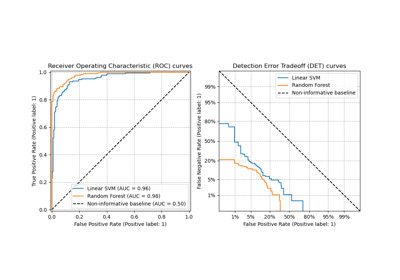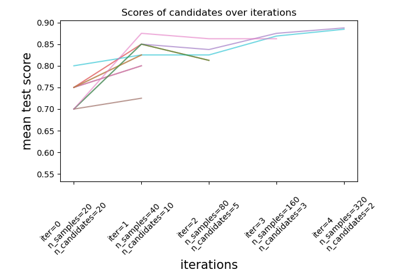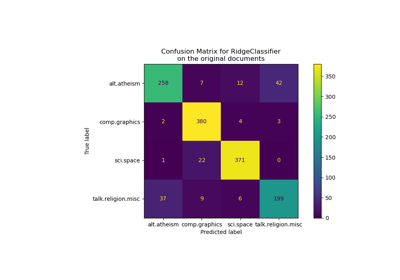# sklearn.ensemble.RandomForestClassifier¶

class sklearn.ensemble.RandomForestClassifier(n_estimators=100, *, criterion='gini', max_depth=None, min_samples_split=2, min_samples_leaf=1, min_weight_fraction_leaf=0.0, max_features='sqrt', max_leaf_nodes=None, min_impurity_decrease=0.0, bootstrap=True, oob_score=False, n_jobs=None, random_state=None, verbose=0, warm_start=False, class_weight=None, ccp_alpha=0.0, max_samples=None)[source]

A random forest classifier.

A random forest is a meta estimator that fits a number of decision tree classifiers on various sub-samples of the dataset and uses averaging to improve the predictive accuracy and control over-fitting. The sub-sample size is controlled with the max_samples parameter if bootstrap=True (default), otherwise the whole dataset is used to build each tree.

Read more in the User Guide.

Parameters:
n_estimatorsint, default=100

The number of trees in the forest.

Changed in version 0.22: The default value of n_estimators changed from 10 to 100 in 0.22.

criterion{“gini”, “entropy”, “log_loss”}, default=”gini”

The function to measure the quality of a split. Supported criteria are “gini” for the Gini impurity and “log_loss” and “entropy” both for the Shannon information gain, see Mathematical formulation. Note: This parameter is tree-specific.

max_depthint, default=None

The maximum depth of the tree. If None, then nodes are expanded until all leaves are pure or until all leaves contain less than min_samples_split samples.

min_samples_splitint or float, default=2

The minimum number of samples required to split an internal node:

• If int, then consider min_samples_split as the minimum number.

• If float, then min_samples_split is a fraction and ceil(min_samples_split * n_samples) are the minimum number of samples for each split.

Changed in version 0.18: Added float values for fractions.

min_samples_leafint or float, default=1

The minimum number of samples required to be at a leaf node. A split point at any depth will only be considered if it leaves at least min_samples_leaf training samples in each of the left and right branches. This may have the effect of smoothing the model, especially in regression.

• If int, then consider min_samples_leaf as the minimum number.

• If float, then min_samples_leaf is a fraction and ceil(min_samples_leaf * n_samples) are the minimum number of samples for each node.

Changed in version 0.18: Added float values for fractions.

min_weight_fraction_leaffloat, default=0.0

The minimum weighted fraction of the sum total of weights (of all the input samples) required to be at a leaf node. Samples have equal weight when sample_weight is not provided.

max_features{“sqrt”, “log2”, None}, int or float, default=”sqrt”

The number of features to consider when looking for the best split:

• If int, then consider max_features features at each split.

• If float, then max_features is a fraction and max(1, int(max_features * n_features_in_)) features are considered at each split.

• If “auto”, then max_features=sqrt(n_features).

• If “sqrt”, then max_features=sqrt(n_features).

• If “log2”, then max_features=log2(n_features).

• If None, then max_features=n_features.

Changed in version 1.1: The default of max_features changed from "auto" to "sqrt".

Deprecated since version 1.1: The "auto" option was deprecated in 1.1 and will be removed in 1.3.

Note: the search for a split does not stop until at least one valid partition of the node samples is found, even if it requires to effectively inspect more than max_features features.

max_leaf_nodesint, default=None

Grow trees with max_leaf_nodes in best-first fashion. Best nodes are defined as relative reduction in impurity. If None then unlimited number of leaf nodes.

min_impurity_decreasefloat, default=0.0

A node will be split if this split induces a decrease of the impurity greater than or equal to this value.

The weighted impurity decrease equation is the following:

N_t / N * (impurity - N_t_R / N_t * right_impurity
- N_t_L / N_t * left_impurity)


where N is the total number of samples, N_t is the number of samples at the current node, N_t_L is the number of samples in the left child, and N_t_R is the number of samples in the right child.

N, N_t, N_t_R and N_t_L all refer to the weighted sum, if sample_weight is passed.

New in version 0.19.

bootstrapbool, default=True

Whether bootstrap samples are used when building trees. If False, the whole dataset is used to build each tree.

oob_scorebool, default=False

Whether to use out-of-bag samples to estimate the generalization score. Only available if bootstrap=True.

n_jobsint, default=None

The number of jobs to run in parallel. fit, predict, decision_path and apply are all parallelized over the trees. None means 1 unless in a joblib.parallel_backend context. -1 means using all processors. See Glossary for more details.

random_stateint, RandomState instance or None, default=None

Controls both the randomness of the bootstrapping of the samples used when building trees (if bootstrap=True) and the sampling of the features to consider when looking for the best split at each node (if max_features < n_features). See Glossary for details.

verboseint, default=0

Controls the verbosity when fitting and predicting.

warm_startbool, default=False

When set to True, reuse the solution of the previous call to fit and add more estimators to the ensemble, otherwise, just fit a whole new forest. See Glossary and Fitting additional weak-learners for details.

class_weight{“balanced”, “balanced_subsample”}, dict or list of dicts, default=None

Weights associated with classes in the form {class_label: weight}. If not given, all classes are supposed to have weight one. For multi-output problems, a list of dicts can be provided in the same order as the columns of y.

Note that for multioutput (including multilabel) weights should be defined for each class of every column in its own dict. For example, for four-class multilabel classification weights should be [{0: 1, 1: 1}, {0: 1, 1: 5}, {0: 1, 1: 1}, {0: 1, 1: 1}] instead of [{1:1}, {2:5}, {3:1}, {4:1}].

The “balanced” mode uses the values of y to automatically adjust weights inversely proportional to class frequencies in the input data as n_samples / (n_classes * np.bincount(y))

The “balanced_subsample” mode is the same as “balanced” except that weights are computed based on the bootstrap sample for every tree grown.

For multi-output, the weights of each column of y will be multiplied.

Note that these weights will be multiplied with sample_weight (passed through the fit method) if sample_weight is specified.

ccp_alphanon-negative float, default=0.0

Complexity parameter used for Minimal Cost-Complexity Pruning. The subtree with the largest cost complexity that is smaller than ccp_alpha will be chosen. By default, no pruning is performed. See Minimal Cost-Complexity Pruning for details.

New in version 0.22.

max_samplesint or float, default=None

If bootstrap is True, the number of samples to draw from X to train each base estimator.

• If None (default), then draw X.shape samples.

• If int, then draw max_samples samples.

• If float, then draw max_samples * X.shape samples. Thus, max_samples should be in the interval (0.0, 1.0].

New in version 0.22.

Attributes:
estimator_DecisionTreeClassifier

The child estimator template used to create the collection of fitted sub-estimators.

New in version 1.2: base_estimator_ was renamed to estimator_.

base_estimator_DecisionTreeClassifier

Estimator used to grow the ensemble.

estimators_list of DecisionTreeClassifier

The collection of fitted sub-estimators.

classes_ndarray of shape (n_classes,) or a list of such arrays

The classes labels (single output problem), or a list of arrays of class labels (multi-output problem).

n_classes_int or list

The number of classes (single output problem), or a list containing the number of classes for each output (multi-output problem).

n_features_in_int

Number of features seen during fit.

New in version 0.24.

feature_names_in_ndarray of shape (n_features_in_,)

Names of features seen during fit. Defined only when X has feature names that are all strings.

New in version 1.0.

n_outputs_int

The number of outputs when fit is performed.

feature_importances_ndarray of shape (n_features,)

The impurity-based feature importances.

oob_score_float

Score of the training dataset obtained using an out-of-bag estimate. This attribute exists only when oob_score is True.

oob_decision_function_ndarray of shape (n_samples, n_classes) or (n_samples, n_classes, n_outputs)

Decision function computed with out-of-bag estimate on the training set. If n_estimators is small it might be possible that a data point was never left out during the bootstrap. In this case, oob_decision_function_ might contain NaN. This attribute exists only when oob_score is True.

sklearn.tree.DecisionTreeClassifier

A decision tree classifier.

sklearn.ensemble.ExtraTreesClassifier

Ensemble of extremely randomized tree classifiers.

Notes

The default values for the parameters controlling the size of the trees (e.g. max_depth, min_samples_leaf, etc.) lead to fully grown and unpruned trees which can potentially be very large on some data sets. To reduce memory consumption, the complexity and size of the trees should be controlled by setting those parameter values.

The features are always randomly permuted at each split. Therefore, the best found split may vary, even with the same training data, max_features=n_features and bootstrap=False, if the improvement of the criterion is identical for several splits enumerated during the search of the best split. To obtain a deterministic behaviour during fitting, random_state has to be fixed.

References


1. Breiman, “Random Forests”, Machine Learning, 45(1), 5-32, 2001.

Examples

>>> from sklearn.ensemble import RandomForestClassifier
>>> from sklearn.datasets import make_classification
>>> X, y = make_classification(n_samples=1000, n_features=4,
...                            n_informative=2, n_redundant=0,
...                            random_state=0, shuffle=False)
>>> clf = RandomForestClassifier(max_depth=2, random_state=0)
>>> clf.fit(X, y)
RandomForestClassifier(...)
>>> print(clf.predict([[0, 0, 0, 0]]))



Methods

 Apply trees in the forest to X, return leaf indices. Return the decision path in the forest. fit(X, y[, sample_weight]) Build a forest of trees from the training set (X, y). get_params([deep]) Get parameters for this estimator. Predict class for X. Predict class log-probabilities for X. Predict class probabilities for X. score(X, y[, sample_weight]) Return the mean accuracy on the given test data and labels. set_params(**params) Set the parameters of this estimator.
apply(X)[source]

Apply trees in the forest to X, return leaf indices.

Parameters:
X{array-like, sparse matrix} of shape (n_samples, n_features)

The input samples. Internally, its dtype will be converted to dtype=np.float32. If a sparse matrix is provided, it will be converted into a sparse csr_matrix.

Returns:
X_leavesndarray of shape (n_samples, n_estimators)

For each datapoint x in X and for each tree in the forest, return the index of the leaf x ends up in.

property base_estimator_

Estimator used to grow the ensemble.

decision_path(X)[source]

Return the decision path in the forest.

New in version 0.18.

Parameters:
X{array-like, sparse matrix} of shape (n_samples, n_features)

The input samples. Internally, its dtype will be converted to dtype=np.float32. If a sparse matrix is provided, it will be converted into a sparse csr_matrix.

Returns:
indicatorsparse matrix of shape (n_samples, n_nodes)

Return a node indicator matrix where non zero elements indicates that the samples goes through the nodes. The matrix is of CSR format.

n_nodes_ptrndarray of shape (n_estimators + 1,)

The columns from indicator[n_nodes_ptr[i]:n_nodes_ptr[i+1]] gives the indicator value for the i-th estimator.

property feature_importances_

The impurity-based feature importances.

The higher, the more important the feature. The importance of a feature is computed as the (normalized) total reduction of the criterion brought by that feature. It is also known as the Gini importance.

Warning: impurity-based feature importances can be misleading for high cardinality features (many unique values). See sklearn.inspection.permutation_importance as an alternative.

Returns:
feature_importances_ndarray of shape (n_features,)

The values of this array sum to 1, unless all trees are single node trees consisting of only the root node, in which case it will be an array of zeros.

fit(X, y, sample_weight=None)[source]

Build a forest of trees from the training set (X, y).

Parameters:
X{array-like, sparse matrix} of shape (n_samples, n_features)

The training input samples. Internally, its dtype will be converted to dtype=np.float32. If a sparse matrix is provided, it will be converted into a sparse csc_matrix.

yarray-like of shape (n_samples,) or (n_samples, n_outputs)

The target values (class labels in classification, real numbers in regression).

sample_weightarray-like of shape (n_samples,), default=None

Sample weights. If None, then samples are equally weighted. Splits that would create child nodes with net zero or negative weight are ignored while searching for a split in each node. In the case of classification, splits are also ignored if they would result in any single class carrying a negative weight in either child node.

Returns:
selfobject

Fitted estimator.

get_params(deep=True)[source]

Get parameters for this estimator.

Parameters:
deepbool, default=True

If True, will return the parameters for this estimator and contained subobjects that are estimators.

Returns:
paramsdict

Parameter names mapped to their values.

predict(X)[source]

Predict class for X.

The predicted class of an input sample is a vote by the trees in the forest, weighted by their probability estimates. That is, the predicted class is the one with highest mean probability estimate across the trees.

Parameters:
X{array-like, sparse matrix} of shape (n_samples, n_features)

The input samples. Internally, its dtype will be converted to dtype=np.float32. If a sparse matrix is provided, it will be converted into a sparse csr_matrix.

Returns:
yndarray of shape (n_samples,) or (n_samples, n_outputs)

The predicted classes.

predict_log_proba(X)[source]

Predict class log-probabilities for X.

The predicted class log-probabilities of an input sample is computed as the log of the mean predicted class probabilities of the trees in the forest.

Parameters:
X{array-like, sparse matrix} of shape (n_samples, n_features)

The input samples. Internally, its dtype will be converted to dtype=np.float32. If a sparse matrix is provided, it will be converted into a sparse csr_matrix.

Returns:
pndarray of shape (n_samples, n_classes), or a list of such arrays

The class probabilities of the input samples. The order of the classes corresponds to that in the attribute classes_.

predict_proba(X)[source]

Predict class probabilities for X.

The predicted class probabilities of an input sample are computed as the mean predicted class probabilities of the trees in the forest. The class probability of a single tree is the fraction of samples of the same class in a leaf.

Parameters:
X{array-like, sparse matrix} of shape (n_samples, n_features)

The input samples. Internally, its dtype will be converted to dtype=np.float32. If a sparse matrix is provided, it will be converted into a sparse csr_matrix.

Returns:
pndarray of shape (n_samples, n_classes), or a list of such arrays

The class probabilities of the input samples. The order of the classes corresponds to that in the attribute classes_.

score(X, y, sample_weight=None)[source]

Return the mean accuracy on the given test data and labels.

In multi-label classification, this is the subset accuracy which is a harsh metric since you require for each sample that each label set be correctly predicted.

Parameters:
Xarray-like of shape (n_samples, n_features)

Test samples.

yarray-like of shape (n_samples,) or (n_samples, n_outputs)

True labels for X.

sample_weightarray-like of shape (n_samples,), default=None

Sample weights.

Returns:
scorefloat

Mean accuracy of self.predict(X) w.r.t. y.

set_params(**params)[source]

Set the parameters of this estimator.

The method works on simple estimators as well as on nested objects (such as Pipeline). The latter have parameters of the form <component>__<parameter> so that it’s possible to update each component of a nested object.

Parameters:
**paramsdict

Estimator parameters.

Returns:
selfestimator instance

Estimator instance.

## Examples using sklearn.ensemble.RandomForestClassifier¶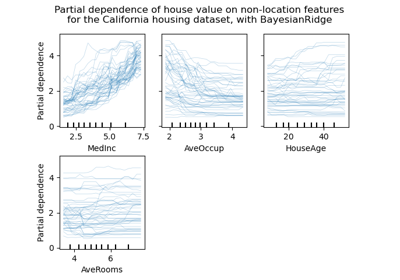Release Highlights for scikit-learn 0.24

Release Highlights for scikit-learn 0.24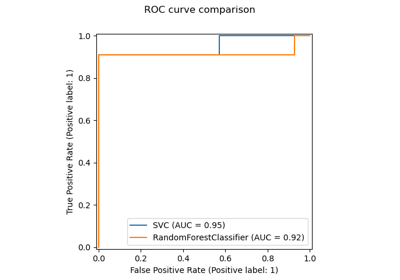Release Highlights for scikit-learn 0.22

Release Highlights for scikit-learn 0.22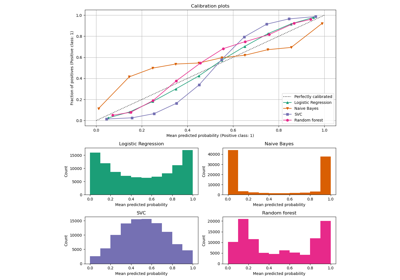Comparison of Calibration of Classifiers

Comparison of Calibration of Classifiers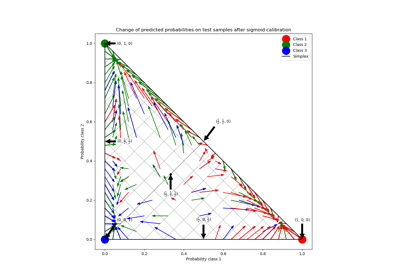Probability Calibration for 3-class classification

Probability Calibration for 3-class classification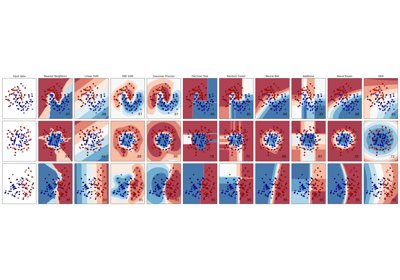Classifier comparison

Classifier comparison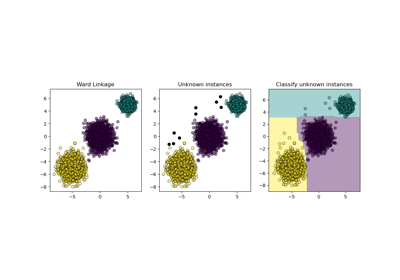Inductive Clustering

Inductive Clustering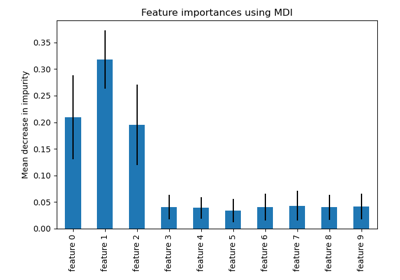Feature importances with a forest of trees

Feature importances with a forest of trees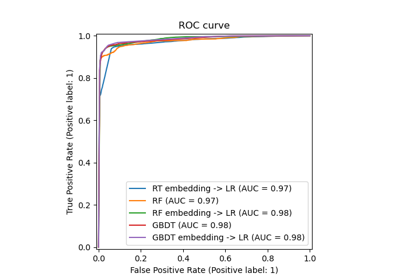Feature transformations with ensembles of trees

Feature transformations with ensembles of trees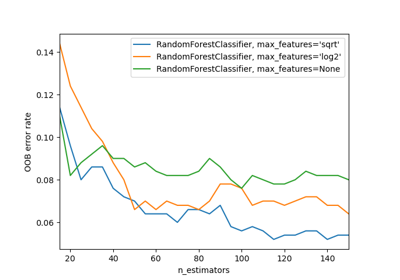OOB Errors for Random Forests

OOB Errors for Random ForestsPixel importances with a parallel forest of trees

Pixel importances with a parallel forest of treesPlot class probabilities calculated by the VotingClassifier

Plot class probabilities calculated by the VotingClassifier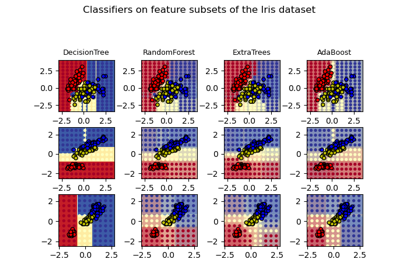Plot the decision surfaces of ensembles of trees on the iris dataset

Plot the decision surfaces of ensembles of trees on the iris dataset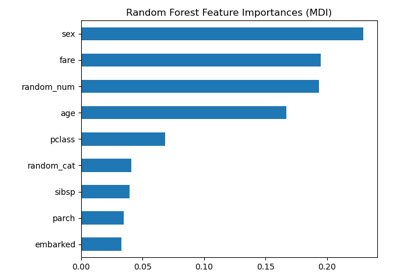Permutation Importance vs Random Forest Feature Importance (MDI)

Permutation Importance vs Random Forest Feature Importance (MDI)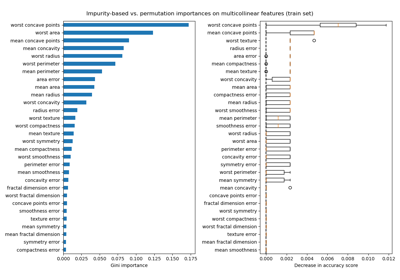Permutation Importance with Multicollinear or Correlated Features

Permutation Importance with Multicollinear or Correlated FeaturesDisplaying Pipelines

Displaying Pipelines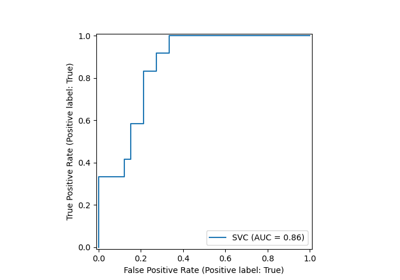ROC Curve with Visualization API

ROC Curve with Visualization API